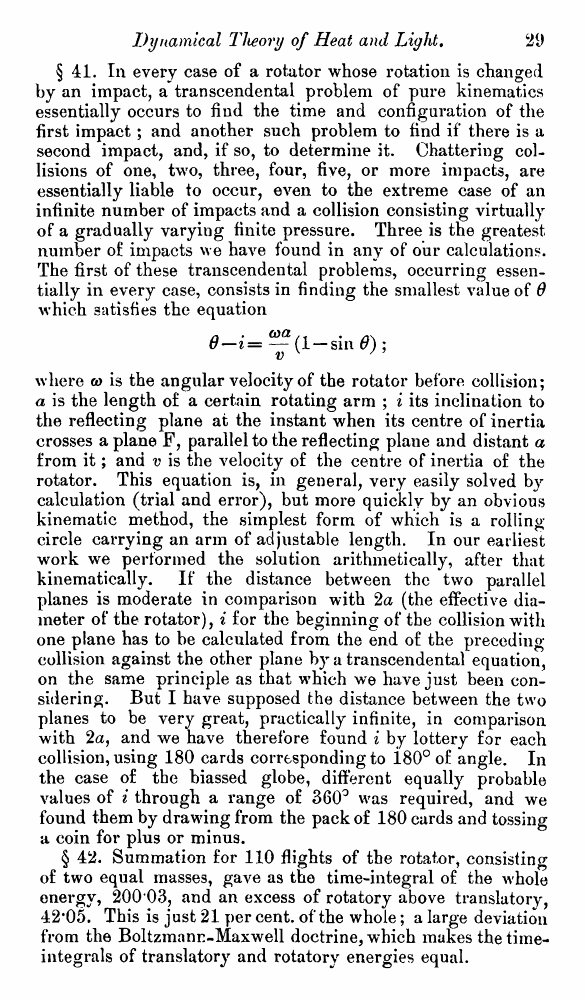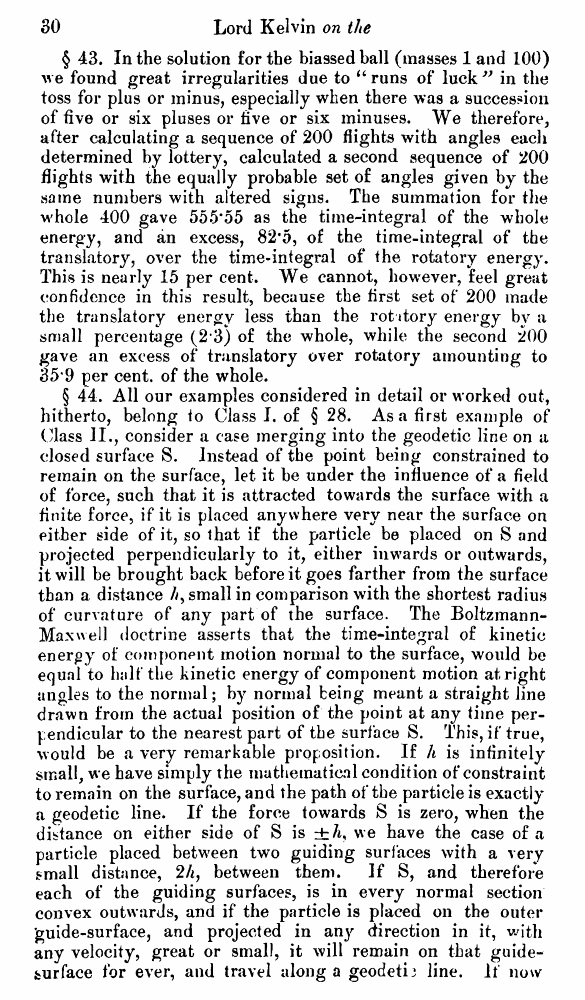﻿ Lord Kelvin. Nineteenth-Century Clouds over the Dynamical Theory of Heat and Light. // Phil. Mag. S. 6. Vol. 2. No. 7. July 1901.

# Lord Kelvin. Nineteenth-Century Clouds over the Dynamical Theory of Heat and Light. // Phil. Mag. S. 6. Vol. 2. No. 7. July 1901.

В начало   Другие форматы   <<<     Страница 29   >>>1  2  3  4  5  6  7  8  9  10  11  12  13  14  15  16  17  18  19  20  21  22  23  24  25  26  27  28  29 30  31  32  33  34  35  36  37  38  39  40 § 41. In every case of a rotator whose rotation is changed by an impact, a transcendental problem of pure kinematics essentially occurs to find the time and configuration of the first impact ; and another such problem to find if there is a second impact, and, if so, to determine it. Chattering collisions of one, twro, three, four, five, or more impacts, are essentially liable to occur, even to the extreme case of an infinite number of impacts and a collision consisting virtually of a gradually varying finite pressure. Three is the greatest number of impacts we have found in any of our calculations. The first of these transcendental problems, occurring essentially in every case, consists in finding the smallest value of 6 which satisfies the equation 6—i= ^(1 — sin 6) ; where co is the angular velocity of the rotator before collision; a is the length of a certain rotating arm ; i its inclination to the reflecting plane at the instant when its centre of inertia crosses a plane F, parallel to the reflecting plane and distant a from it; and v is the velocity of the centre of inertia of the rotator. This equation is, in general, very easily solved by calculation (trial and error), but more quickly by an obvious kinematic method, the simplest form of which is a rolling circle carrying an arm of adjustable length. In our earliest work we performed the solution arithmetically, after that kinematically. If the distance between the two parallel planes is moderate in comparison with 2a (the effective diameter of the rotator), i for the beginning of the collision with one plane has to be calculated from the end of the preceding collision against the other plane by a transcendental equation, on the same principle as that which we have just been considering. But I have supposed the distance between the two planes to be very great, practically infinite, in comparison with 2a, and we have therefore found i by lottery for each collision, using 180 cards corresponding to i80° of angle. In the case of the biassed globe, different equally probable values of i through a range of 360° was required, and we found them by drawing from the pack of 180 cards and tossing a coin for plus or minus. § 42. Summation for 110 flights of the rotator, consisting of two equal masses, gave as the time-integral of the whole energy, 200 03, and an excess of rotatory above translatory, 42*05. This is just 21 per cent, of the whole; a large deviation from the Boltzmann-Maxwell doctrine, which makes the time-integrals of translatory and rotatory energies equal. § 43. In the solution for the biassed ball (masses 1 and 100) we found great irregularities due to “ runs of luck” in the toss for plus or minus, especially when there was a succession of five or six pluses or five or six minuses. We therefore, after calculating a sequence of 200 flights with angles each determined by lottery, calculated a second sequence of 200 flights with the equally probable set o£ angles given by the same numbers with altered signs. The summation for the whole 400 gave 555*55 as the time-integral of the whole energy, and an excess, 82*5, of the time-integral of the translatory, over the time-integral of the rotatory energy. This is nearly 15 per cent. We cannot, however, feel great confidence in this result, because the first set of 200 made the translatory energy less than the rot itory energy by a small percentage (2 3) of the whole, while the second 200 gave an excess of translatory over rotatory amounting to 35*9 per cent, of the whole. § 44. All our examples considered in detail or worked out, hitherto, belong to Class I. of § 28. Asa first example of ("lass II., consider a case merging into the geodetic line on a closed surface S. Instead of the point being constrained to remain on the surface, let it be under the influence of a field of force, such that it is attracted towards the surface with a finite force, if it is placed anywhere very near the surface on either side of it, so that if the particle be placed on S and projected perpendicularly to it, either inwards or outwards, it will be brought back before it goes farther from the surface than a distance //, small in comparison with the shortest radius of curvature of any part of the surface. The Boltzmann-Maxwell doctrine asserts that the time-integral of kinetic energy of component motion normal to the surface, would be equal to half the kinetic energy of component motion at right angles to the normal; by normal being meant a straight line drawn from the actual position of the point at any time perpendicular to the nearest part of the surface S. This, if true, would be a very remarkable proposition. If h is infinitely small, we have simply the mathematical condition of constraint to remain on the surface, and the path of the particle is exactly a geodetic line. If the force towards S is zero, when the distance on either side of S is we have the case of a particle placed between two guiding surfaces with a very small distance, 2A, between them. If S, and therefore each of the guiding surfaces, is in every normal section convex outwards, and if the particle is placed on the outer 'guide-surface, and projected in any direction in it, with any velocity, great or small, it will remain on that guide-jburface for ever, and travel along a geodetij line. If now